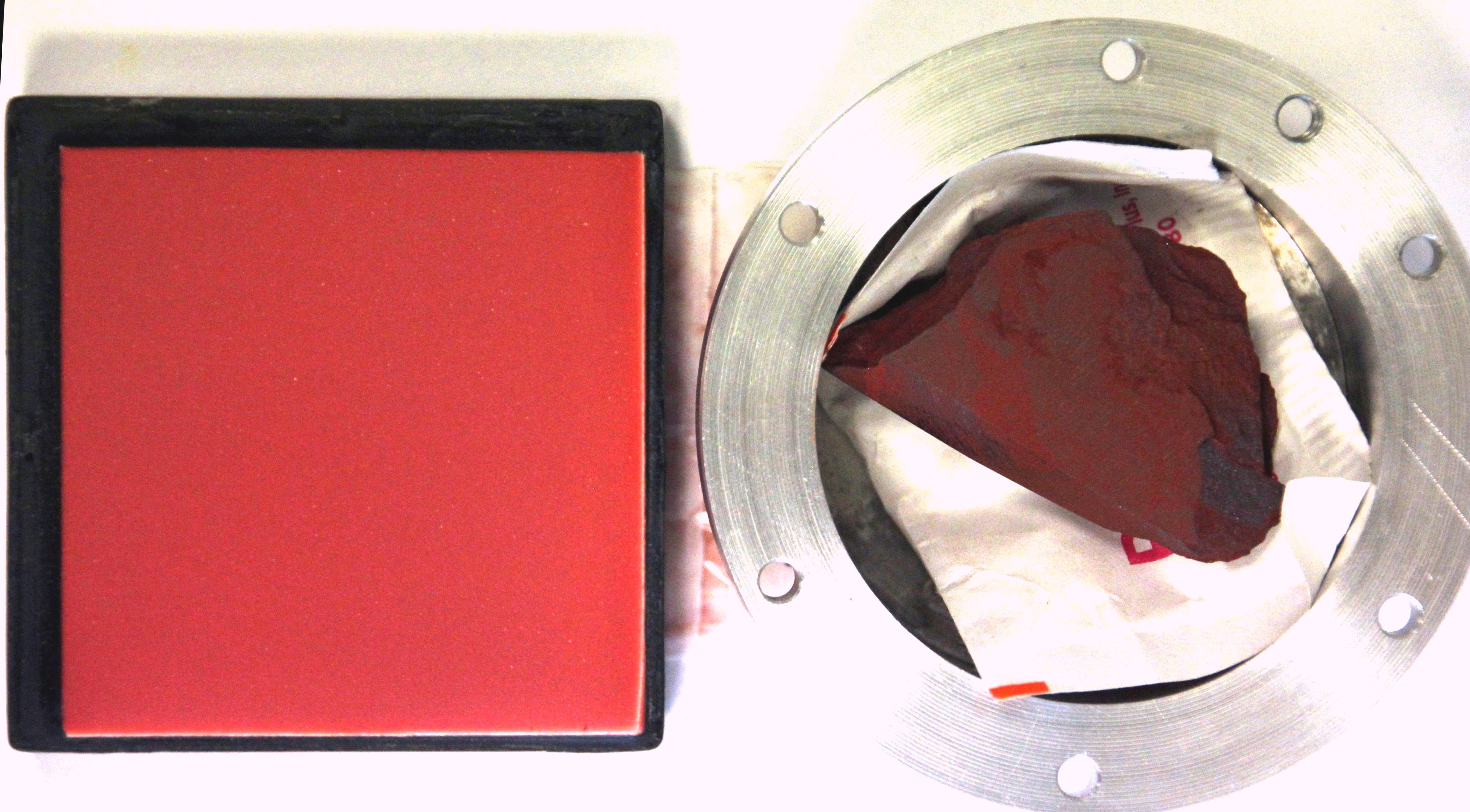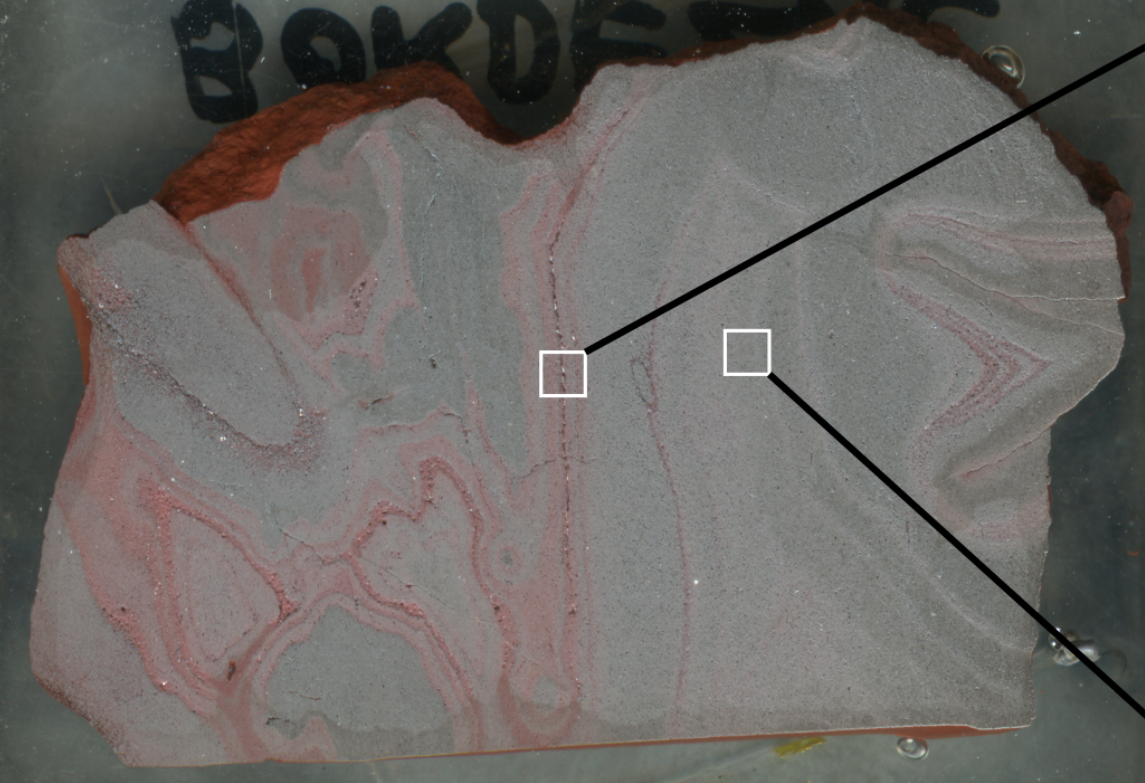Matter
Name
Pigment Bordezac (PIG-0160-2016-D), facies D, hematite-rich vein
Family
mineral
Database
Experimentalists
Level
from laboratory repository
Origins
natural terrestrial
Provider
Pigmenthothèque - EDYTEM
Provider reference code
Bordezac – PIG-0160-2016-D (2016-027)
Local reference code
Pig-0160-2016-D_Bordezac
Body name
Earth
Terrain type
mineral surface
Place
Road D51, SE Bordezac (Gard) , Occitanie , France

#### Coordinates

System
WGS84
Position type
point
Geolocation
Point Latitude Longitude Altitude
#1 44.3104° 4.0761°Preparation
raw
Storage condition
room temperature and conditions
Phase type
solid
Component type (group)
sedimentary rock (iron-sediment or ironstone)
Hematite-rich vein - Hydrothermal mineralization in an arkosic sandstone bank - Era: Mesozoic, Trias, Trias series, inferior (?) and middle trias level
Shape
Irregular shaped red-bordeaux block with grey-red patinated faces and the other are light brown inlaid sides
Texture
compact fine grained
Type of porosity
without pores
Texture
compact
Grain sizes
Grains Size min Size max Size median Size width Size mass fraction Grain shape
#1 0.0 ${\mu}m$ 200.0 ${\mu}m$ None
Size distribution
hematite (>50%): < ? µm, muscovite (5%): 100-200µm platelet; quartz (5%): < 100µm, Xenotime (<1%): 5µm, Baryte: ?)
types of shape: equant/spherical, tabular (muscovite)
Oxides
 Formula Mass fraction (%) $SiO_{2}$ $Al_{2}O_{3}$ $Fe_{2}O_{3}$ $MnO$ $MgO$ $CaO$ $Na_{2}O$ $K_{2}O$ $TiO_{2}$ $P_{2}O_{5}$ 13.56 6.73 74.79 0.083 0.34 0.07 0.015 1.96 0.18 0.14
Contain K (1.96% in oxide) and small impurity of P, Ti, Na, Ca, Mg, Mn (0.83% in oxides), fire loss: 2.3%
Number
5
Mode of mixing
homogeneous mixing
Materials
Name Arrangement Mass (g) Mass fraction Abundance comments
Quartz grains embeded in a ferric matrix (hematite), mostly in cracks, sometimes isolated 0.05 assumed mass fraction = cross section fraction. Impurity abundances not determined
tabular muscovite grains embeded in a ferric matrix (hematite) 0.04 $\pm$ 0.01 < 5%. Assumed mass fraction = cross section fraction
Xenotime-(Y) grains embeded in a ferric matrix (hematite), mostly in cracks 0.01 <1%. Assumed mass fraction = cross section fraction
Baryte grains embeded in a ferric matrix (hematite), mostly in cracks, sometimes isolated <1%. Assumed mass fraction = cross section fraction
Hematite ferric matrix of hematite containing muscovite, quartz, xenotime and baryte grains) > 50%
Documentations